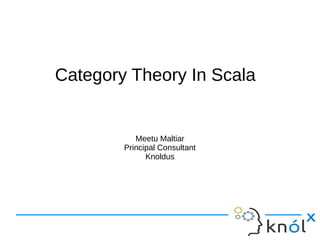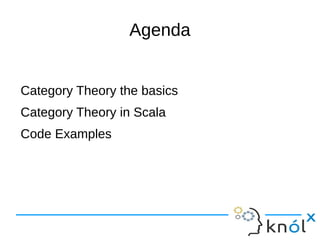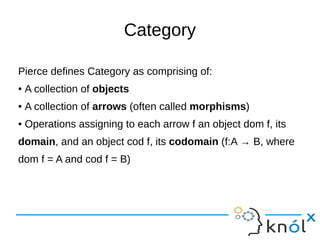Se está descargando tu SlideShare. ×

# Scala categorytheory

Anuncio
Anuncio
Anuncio
Anuncio
Anuncio
Anuncio
Anuncio
Anuncio
Anuncio
Anuncio
AnuncioCargando en…3
×

1 de 22 Anuncio

# Scala categorytheory

Category theory in scala. This deals with basics of Category Theory and its relation with Scala.

Category theory in scala. This deals with basics of Category Theory and its relation with Scala.

Anuncio
Anuncio

### Scala categorytheory

1. 1. Category Theory In Scala Meetu Maltiar Principal Consultant Knoldus
2. 2. Agenda Category Theory the basics Category Theory in Scala Code Examples
3. 3. Category Pierce defines Category as comprising of: ● A collection of objects ● A collection of arrows (often called morphisms) ● Operations assigning to each arrow f an object dom f, its domain, and an object cod f, its codomain (f:A → B, where dom f = A and cod f = B)
4. 4. Category continued.. A composition operator assigning to each pair of arrows f and g with cod f = dom g, a composite arrow g o f: dom f → cod g, satisfying the following associative law: for any arrows f:A → B, g:B → C and h:C → D h o (g o f) = (h o g) o f For each object A, an identity arrow idA: A → A satisfying the following identity law: for any arrow f:A → B, idB o f = f and f o idA = f
5. 5. Category Apples Fruit Banana Vegetable Brinjal f: A → B
6. 6. Category Composition Apples Fruit Living Banana Vegetable Non Living Brinjal g o f: A → C
7. 7. Translating to Scala We can define any function from a type A to type B as: A => B here we have an example or morphism For any function val foo: A => B = //Anything We have type A as domain of function foo and type B as co-domain of function foo
8. 8. Scala Category Example We can define composition of arrows or functions in Scala with following REPL example scala> val f: Int => String = _.toString f: Int => String = <function1> scala> val g: String => Int = _.length g: String => Int = <function1> scala> f compose g res3: String => String = <function1>
9. 9. Scala category identity continued Identity law is a special version of a composition. Let's define function and play them in REPL: scala> val foo: Int => String = _.toString foo: Int => String = <function1> scala> val idInt: Int => Int = identity(_: Int) idInt: Int => Int = <function1> scala> val idString: String => String = identity(_: String) idString: String => String = <function1> scala> idString compose foo res4: Int => String = <function1> scala> foo compose idInt res5: Int => String = <function1>
10. 10. Category theory and PL Do understanding Category Theory makes us understand PL's better? If we just do Enterprise software development and do not want to go beyond our comfort zone then answer is no. Category Theory provides uniform model of set theory, algebra, logic and computation. Many concepts of Category theory maps nicely to structures of PL. Categorical reasoning helps us to reason about programs. Some basic structures in PL like product and sum types have their correspondences in Category Theory. There is a strong correspondence between typed lambda calculus and cartesian closed categories
11. 11. Properties of data type in CT Consider the category of Products of elements. Take for example of cartesian products from the category of sets. A cartesian product of two sets A and B is defined by A X B = {(a, b) | a belongs to A and b belongs to B} For example if A = {1, 2} and B = {A, B} A X B = {(1, A), (1, B), (2, A), (2, B)} So here we have tuples or Pairs as objects in a Category
12. 12. Properties of data type in CT .. But what will be morphisms? In case of products, the applicable arrows (or morphisms) are the projection functions: π1: A X B → A π1: A X B → B
13. 13. Properties of data type in CT .. Now if we draw a Category diagram with C as a product type we have two functions as projection functions: 1. f: C → A 2. g: C → B and the product function is represented by: C → A X B It is defined by: <F, G>(x) = (f(x), g(x))
14. 14. Diagram of CT C f g ! [f,g] A AXB B π1 π2
15. 15. Diagram of CT For every pair of vertices X and Y, all paths in the diagram from X to Y are equal in the sense that each path forms an arrow and these arrows are equal in category For example the path from C to A is: <f,g> and π1 therefore composition gives us: π1 o <f,g> = f Also path from C to B gives us: <f,g> and π2 π2 o <f,g> = g
16. 16. Scala CT example Lets now see how the laws of commutativity maps to Scala. As a programmer we use the projection functions (_1 and _2) in Scala Tuple2 on a regular basis In the CT diagram we will see that we get additional insights in abstraction and help understand mathematical properties of how cartesian product of sets map translates to composition of functions
17. 17. Scala CT example... scala> val ip = (10, "meetu") ip: (Int, java.lang.String) = (10,meetu) scala> val pi1: ((Int, String)) => Int = (p => p._1) pi1: ((Int, String)) => Int = <function1> scala> val pi2: ((Int, String)) => String = (p => p._2) pi2: ((Int, String)) => String = <function1> scala> val f: Int => Int = (_ * 2) f: Int => Int = <function1> scala> val g: Int => String = _.toString g: Int => String = <function1> scala> val `<f, g>`: Int => (Int, String) = (x => (f(x), g(x))) <f, g>: Int => (Int, String) = <function1> scala> pi1 compose `<f, g>` res0: Int => Int = <function1> scala> pi2 compose `<f, g>` res1: Int => String = <function1>
18. 18. Scala CT example So we can claim from the commutativity of the diagram that: pi1 compose `<f, g>` is type wise equal to f pi2 compose `<f, g>` is type wise equal to g Category theory says that morphism between C and A X B is unique and that A X B is defined upto isomorphism. Uniqueness is denoted by ! In diagram. This makes sense as well because a pair can be unique
19. 19. Interface driven modeling Category Theory maps very closely to PL in the sense that it focuses on arrows rather than objects corresponding to Interfaces Pierce: CT typically “abstracts away from elements, treating objects as black boxes with unimagined internal structure and focusing attention on the properties of arrows between objects” Learning CT enriches both CT and PL. For example if we know what a Functor is in CT then we can easily make it generic enough so that it can interact with other Functors
20. 20. Thinking Generically CT talks about objects and morphisms and how arrows compose. A special kind of morphism is identity function in programming. It is 0 in addition and 1 in multiplication CT generalizes this concept by using same vocabulary (morphism) to denote both stuff that does some operations and those that don't For every object X, there exists a morphism idX: X → X called identity morphism on X, such that for every morphism f: A → B we have: IdB o f = f = f o idA (used in monoids)
21. 21. Duality We have seen example of SumTypes for Product. If we look at the CT diagram of Sum compared to Product we see that arrows are reversed. This is called a dual in CT. In Scala we model it by a Union Type like Either where value of SumType comes either from left or right
22. 22. What's Next Functors Monads Scalaz Resources: 1. Debasish Ghosh blog “Does Category Theory Makes you A Better Programmer” 2. Heiko's blog on Category Theory 3. Runar video on Scalaz